﻿ 地球同步轨道圆迹SAR研究
«上一篇文章快速检索 高级检索

 雷达学报2015, Vol. 4Issue (3): 241–253  DOI: 10.12000/JR150620

### 引用本文 [复制中英文]

[复制中文]
Hong Wen, Lin Yun, Tan Wei-xian, Wang Yan-ping, Xiang Mao-sheng. Study on Geosynchronous Circular SAR. Journal of Radars, 2015, 4(3): 241–253. DOI: 10.12000/JR15062.
[复制英文]

### 文章历史, , , ,

Study on Geosynchronous Circular SAR, , , ,
Institute of Electronics, Chinese Academy of Sciences, Beijing 100190, China
Abstract: The concept of Geosynchronous Circular SAR (Geo-CSAR) is introduced in this paper. With the design of the geosynchronous orbit parameters, a near-circular satellite sub-track could be formed to enable the staring imaging mode, which supports the advanced applications for wide-field and 3-D information acquisition under long-term consistent observation. This paper also analyzes Geo-CSAR’s imaging formation capabilities, and concludes its attractive advantages over low-earth orbit spaceborne SAR in terms of instantaneous coverage, consistent observing area, 3-D positioning accuracy and etc.. Encouraging expectations for Geo-CSAR thus could be positively predicted in military investigation and disaster monitoring management applications.
Key words: Earth observation    Geosynchronous orbit    Synthetic Aperture Radar (SAR)     Circular SAR (CSAR)     Wide-field consistent observation     3-D imaging
1 引言

1978年，第1颗星载SAR SEASAT发射成功，以25 m的分辨率对地球表面一亿两千万平方公里的面积进行了测绘，获得了从未有过的陆地、海洋和冰川等数据，开辟了SAR对地观测的新时代。经过几十年的迅猛发展，星载SAR技术日趋成熟，在轨和在研的星载SAR多已具备高分辨率、多极化、多波段、多工作模式等能力。

(1) 瞬时覆盖度小，重访时间长，难以实现对热点区域的快速响应

(2) 波束覆盖面积小，过顶时间短，难以实现大面积区域的连续监测

(3) 只能获取2维图像，难以实现目标的精确定位和打击

2 研究背景 2.1 圆迹SAR简介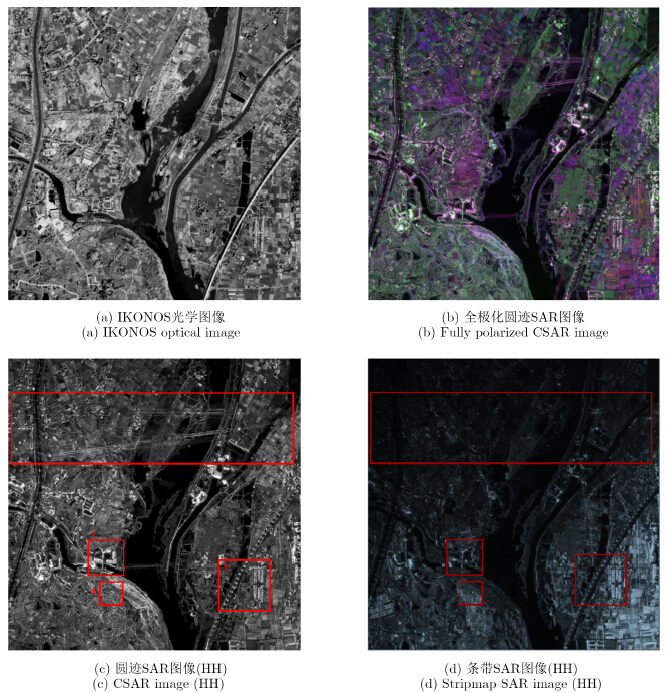图 1 2011年8月中科院电子所获取了P波段360°全方位高分辨圆迹SAR图像 Fig.1 P band 360° full-aspect high resolution CSAR image obtained by IECAS on Aug. 2011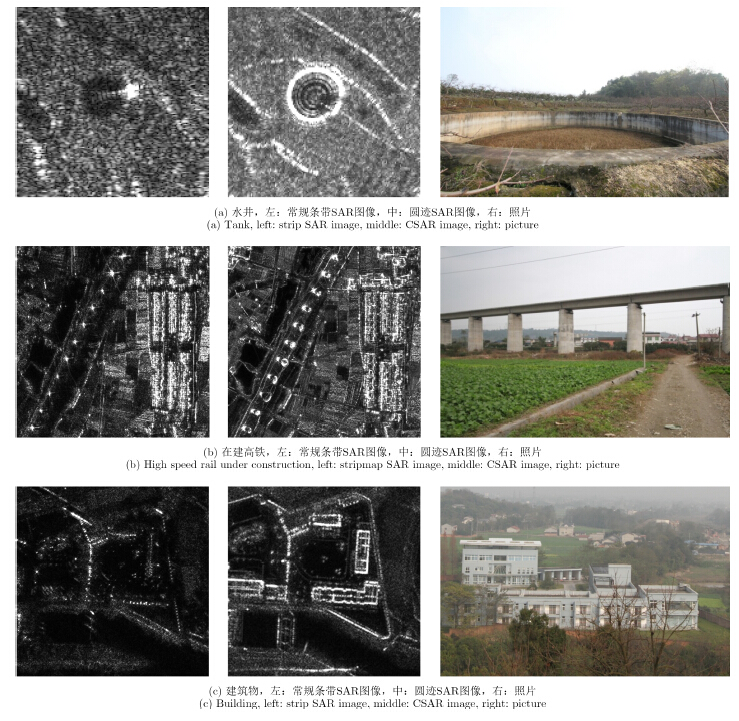图 2 圆迹SAR图像与常规条带SAR图像的细节对比(HH) Fig.2 Detailed comparison between CSAR images and stripmap SAR images (HH)

2.2 地球同步轨道SAR简介

1978年，K. Tomiyasu首次提出了地球同步轨道SAR(Geosynchronous SAR,GeoSAR)的概念，随后初步设计了轨道倾角为50°的GeoSAR系统，该系统可将美国本土全境观测时间缩短为3 h，观测周期缩短为1 d。受当时天线工艺、发射功率和运载能力等技术因素限制，GeoSAR在很长一段时间仅停留在概念研究阶段。图 3 美国GESS计划的GEOSAR 组网卫星观测能力 Fig.3 The imaging capability of the American GESS GEOCSAR constellation

3 地球同步轨道圆迹SAR的概念

3.1 轨道生成

 $\begin{array}{l} {J_s} = 2\left( {\hat n \times {H^i}} \right);{H_s} = 0\\ {J_s} = 0 \end{array}$ (1)

 $A = \sqrt{{\mu /{\psi ^2}}} = 42164.2{\rm{km}}$ (2)

“8”字型卫星轨迹是通过大倾角圆形同步轨道形成的，Geo-CSAR的轨迹生成机理则很不同，是在地球静止轨道(地球静止轨道同时满足a=Ai=e=0)的基础上，通过设计较小的偏心率和倾角来实现近似圆形的卫星轨迹。设轨道半径误差为δa=a-A，则当i,e和δa都较小时，式(1)可近似表达为
 $\left. {\begin{array}{*{20}{l}} {r \approx A + \delta a - Ae\cos \upsilon }\\ {\lambda \approx {\lambda _{\rm{0}}} - 1.5 \cdot (\delta a/A) \cdot \psi t + 2e\sin \upsilon }\\ {\varphi \approx i\sin (\omega + \upsilon )} \end{array}} \right\}$ (3)

 ${\lambda _{\rm{0}}}{\rm{ = }}\Omega - G + \arg \left[{\cos \omega + {\rm{j}}\sin \omega \cdot \cos i} \right]$ (4)

 $\left. {\begin{array}{*{20}{l}} {\delta a = 0}\\ {\omega {\rm{ = }}\pi {\rm{/2}}{\rm{3}}\pi {\rm{/2}}}\\ {i = 2e} \end{array}} \right\}$ (5)

 $\left. {\begin{array}{*{20}{l}} {r \approx A - Ae\cos \upsilon }\\ {\lambda \approx {\lambda _{\rm{0}}} + 2e\sin \upsilon }\\ {\varphi \approx i\cos \upsilon {\rm{ - }}i\cos \upsilon } \end{array}} \right\}$ (6)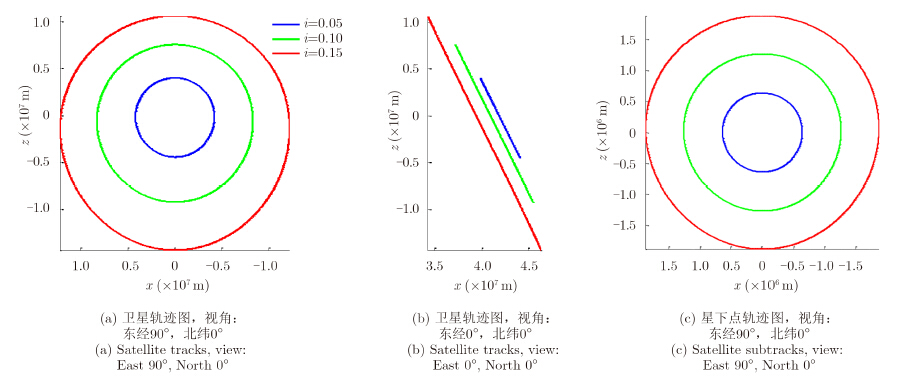图 4 不同轨道参数下的Geo-CSAR卫星轨迹Fig. 4 Geo-CSAR satellite tracks with different orbit parameters
3.2 分辨率理论

Geo-CSAR可实现2维和3维两种成像模式：2维成像模式下，全孔径划分为若干弯曲度可忽略的小角度孔径，小角度孔径近似为直线，合成后可获得可视范围内目标区域的2维图像，也可进一步形成同一目标区域的时间序列2维图像，实现对热点区域的动态监视；3维成像模式下，24 h全孔径合成，因天线相对地面目标的观测视角变化较小(约为2i，通常不超过30°)，可以认为目标的散射特性在整个孔径内保持恒定，能够获得可视范围内目标区域的高分辨3维图像，实现重点区域的精细观测。本小节将讨论Geo-CSAR的2维和3维分辨率理论。

3.2.1 2维分辨率

 ${\rho _{\rm{r}}} = \frac{{\rm{c}}}{{2B}}$ (7)

 ${\rho _{\rm{a}}} = \frac{{{\lambda _{\rm{c}}}}}{{4\sin \dfrac{{{\theta _{{\rm{syn}}}}}}{2}}}$ (8)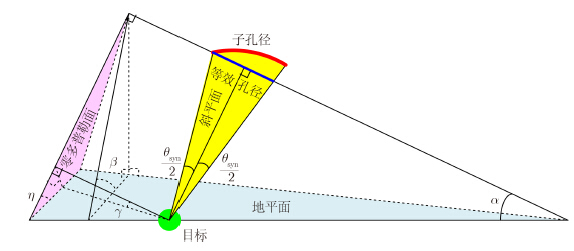图 5 小角度孔径成像几何Fig. 5 Small angle aperture imaging geometry

 ${\rho _{{\rm{rg}}}} = \frac{{{\rho _{\rm{r}}}}}{{\cos \eta }}$ (9)

 $\cos \eta = \frac{{\cos \beta }}{{\sqrt {{{\sin }^2}\alpha {{\cos }^2}\beta + {{\cos }^2}\alpha } }}$ (10)

 ${\rho _{{\rm{ag}}}} = \frac{{{\rho _{\rm{a}}}}}{{\cos \gamma }}$ (11)

 $\cos \gamma = \frac{1}{{\sqrt {1 + {{\tan }^2}\alpha {{\tan }^2}\eta } }}$ (12)
3.2.2 维分辨率

 $\left. {\begin{array}{*{20}{l}} {{k_x} = \frac{{\partial \Phi }}{{\partial {x_{\rm{a}}}}} = - k\frac{{{x_{\rm{a}}} - {x_{\rm{p}}}}}{{{R_{\rm{p}}}}}}\\ {{k_y} = \frac{{\partial {\rm{ }}\Phi }}{{\partial {y_{\rm{a}}}}} = - k\frac{{{y_{\rm{a}}} - {y_{\rm{p}}}}}{{{R_{\rm{p}}}}}}\\ {{k_z} = \frac{{\partial {\rm{ }}\Phi }}{{\partial {z_{\rm{a}}}}} = - k\frac{{{z_{\rm{a}}} - {z_{\rm{p}}}}}{{{R_{\rm{p}}}}}} \end{array}} \right\}$ (13)

 $phi = - k{R_{\rm{p}}} = - k\sqrt {{{({x_{\rm{a}}} - {x_{\rm{p}}})}^2} + {{({y_{\rm{a}}} - {y_{\rm{p}}})}^2} + {{({z_{\rm{a}}} - {z_{\rm{p}}})}^2}}$ (14)
k=4πƒ/c为双程波数，f为发射的宽带信号频率，Rp为卫星到目标的距离，(xa,ya,za)为卫星坐标，(xp,yp,zp)为目标坐标。根据式(13)，可得到如图 7所示的波数域频谱支撑域，支撑域近似为一个圆台曲面，具有3维结构，证明了Geo-CSAR的3维分辨能力。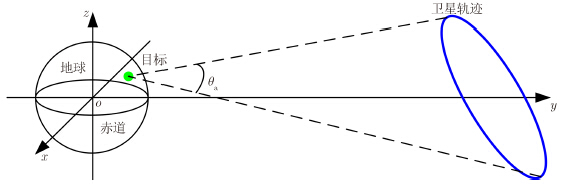图 6 全孔径成像几何Fig. 6 Full aperture imaging geometry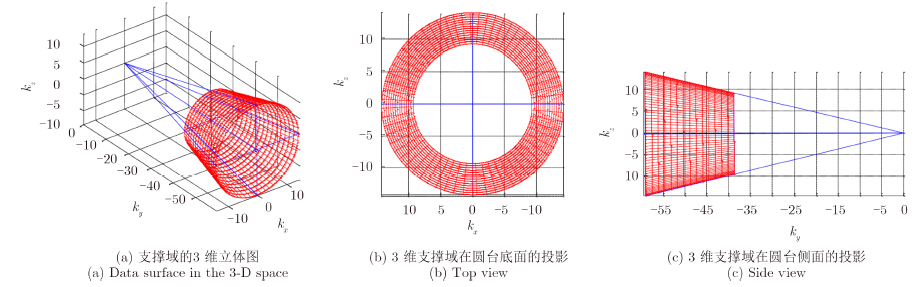图 7 点目标3维频谱支撑域Fig. 7 Data surface observed in the 3-D frequency space

3维频谱在圆台底面的投影近似为一个中空的圆环，圆环外径为：
 ${B_{\rm{a}}} = {k_{\max }}\sin {\theta _{\rm{a}}}$ (15)

 ${\theta _{\rm{a}}} \approx 2i$ (16)

 ${\rho _{\rm{a}}} \approx \frac{{{\rm{2}}\pi }}{{{B_{\rm{a}}}}} = \frac{{{\lambda _{\min }}}}{{4\sin ({\theta _{\rm{a}}}/2)}} \approx \frac{{{\lambda _{\min }}}}{{{\rm{4}}\sin i}}$ (17)

 ${B_{\rm{h}}} = \frac{{4\pi B}}{{\rm{c}}}\cos ({\theta _{\rm{a}}}/2) \approx \frac{{4\pi B}}{{\rm{c}}}\cos i$ (18)

 ${\rho _{\rm{h}}} = \frac{{2\pi }}{{{B_{\rm{h}}}}} = \frac{{\rm{c}}}{{2B\cos ({\theta _{\rm{a}}}/2)}} \approx \frac{{\rm{c}}}{{2B\cos i}}$ (19)
4 成像能力表 1 轨道参数和系统参数 Tab. 1 Orbital parameters and system parameters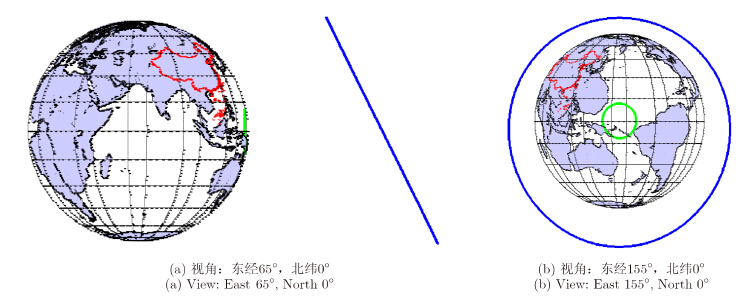图 8 卫星轨迹Fig. 8 Satellite track

4.1 单颗卫星的成像能力

(1) 2维成像能力

 ${\theta _{{\rm{syn}}}} \approx 2{\rm{arcsin}}\left[ {\frac{{A\sin i\sin (2\pi /48/2)}}{{A - {R_{\rm{e}}}}}} \right] = 1.75^\circ {\rm{ }}$ (20)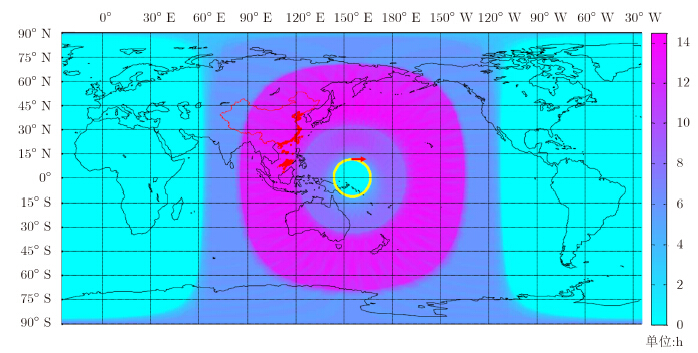图 9 单颗卫星时，达到2维成像指标要求的地球表面时间覆盖度Fig. 9 The time coverage of the land surface in view for a single satellite with the given 2-D imaging performance requirements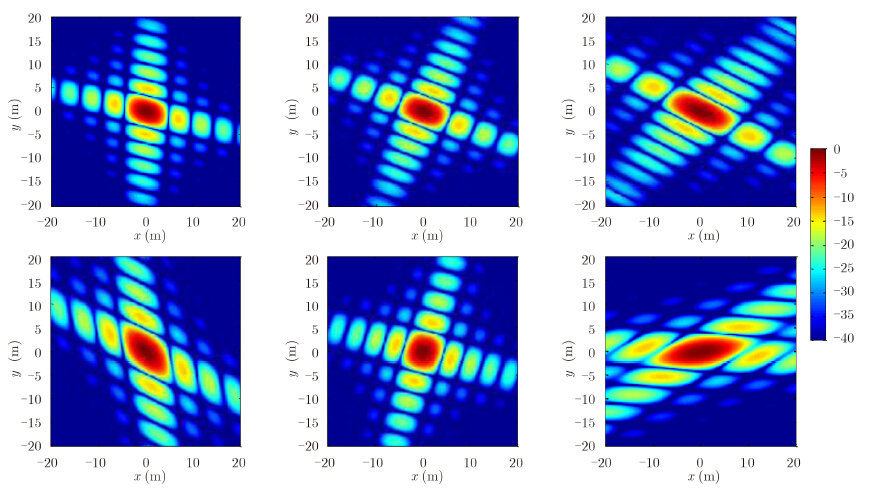图 10 Geo-CSAR 2维成像模式下，位于东经110°，北纬19°(海南)的点目标的点扩展函数(从左至右分别为第10,15,20,36,41和46幅序列图像)Fig. 10 The spread function of the point target located at East 110°,North 19° (Hainan) of the Geo-CSAR 2-D imaging mode. (The sequences are 10,15,20,36,41,46 respectively)

(2) 3维成像能力

3维成像模式下，24 h全孔径合成，全孔径相对于地面目标的视角变化约为2i，本参数下，视角变化约为23°，可以认为目标的散射特性在整个孔径内保持恒定，具备真3维成像的条件。因全孔径相对于地面不同位置处的目标视角变化范围差别不大，则3维分辨率的空变性也不大，根据式(17)和式(19)计算得到：平面分辨率约为0.33 m，高程分辨率约为0.3 m，本文以此作为3维成像模式的指标。图 11给出了满足指标要求的覆盖区域(紫色)，覆盖率为地球总面积的33%。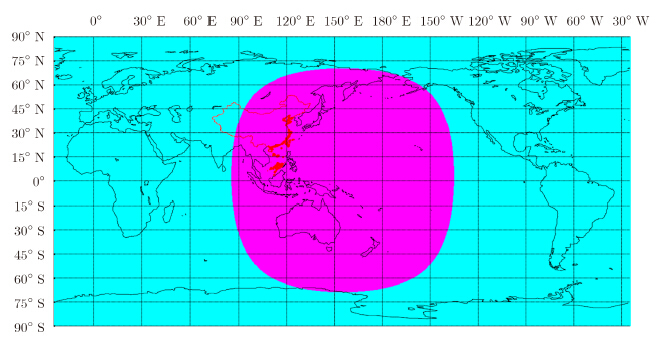图 11 单颗卫星时，3维成像覆盖区域Fig. 11 The 3-D imaging land surface coverage for a single satellite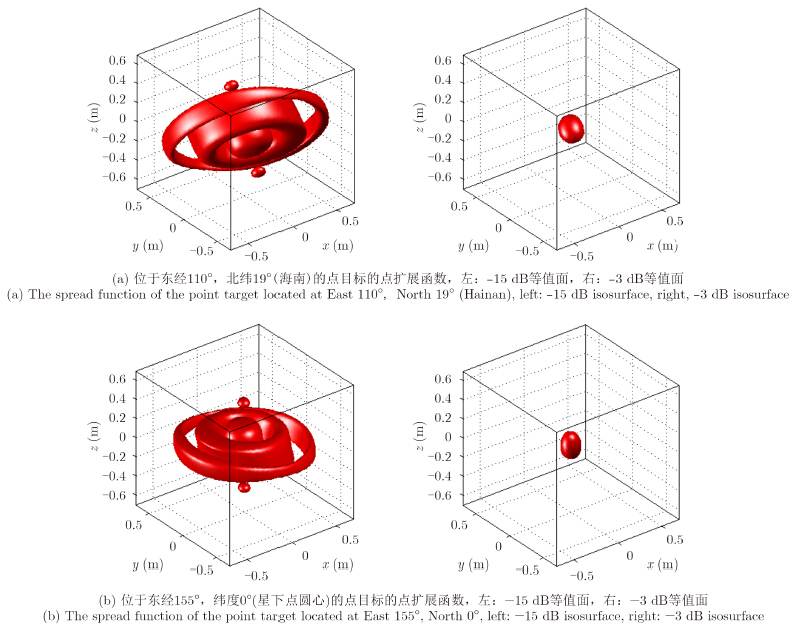图 12 Geo-CSAR 3维成像模式下的点扩展函数Fig. 12 The point target spread function of the Geo-CSAR 3-D imaging mode
4.2 Geo-CSAR星座的成像能力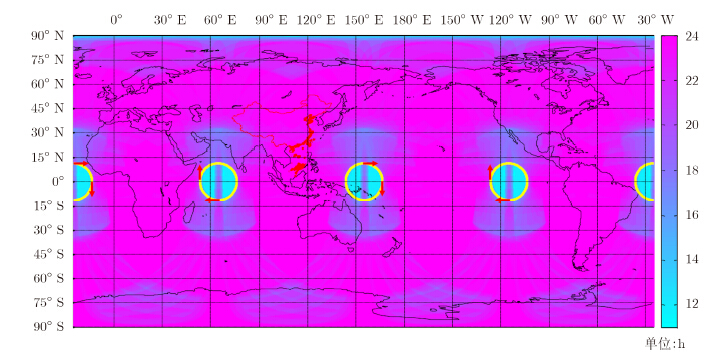图 13 8颗卫星协同工作时，达到指标要求的地球表面时间覆盖度Fig. 13 The time coverage of the land surface in view for a constellation of 8 satellites with the given imaging performance requirments

3维成像模式下，可在每组卫星中任选1颗，共4颗，对4颗卫星的24 h可视范围进行叠加，得到如图 14所示的3维成像覆盖区域(紫色)，覆盖率达到地球表面总面积的92%，非覆盖区(青色)处于两极。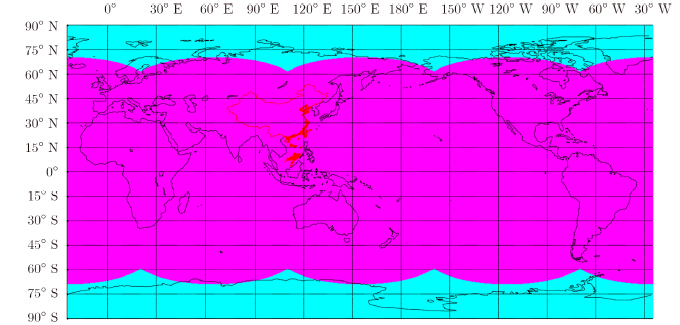图 14 3维成像覆盖区域(紫色区域)Fig. 14 3-D imaging land surface coverage (Purple area)

5 结束语

Geo-CSAR的实现目前还面临着理论和技术上的挑战，除了系统代价高、受电离层影响大等GeoSAR的共性问题外，Geo-CSAR的研究难点主要包括以下两个方面：(1)Geo-CSAR的合成孔径时间较长(3维成像时达到24 h)，对轨道测量手段的稳定性和精度都提出了极高的要求。因此，长合成孔径时间条件下Geo-CSAR的高精度定轨和运动补偿问题有待研究和突破。(2)Geo-CSAR成像区域面积大、合成孔径时间长、分辨率高，将不仅产生海量数据问题，还使地球的球面性以及卫星轨迹的非规则性等因素变得不可忽视，这给成像处理，尤其是3维成像处理，带来了巨大挑战，亟需开展Geo-CSAR的高精度大区域快速成像算法研究。

  Battagliere M L. The COSMO-SkyMed experience overview[OL]. https://wiki.services.eoportal.org/.../COSMOSkyMed_ Experience_WORKSHOP_ESRIN_Oct_2012_ FinalVersion.ppt.(1)  Zhu X and Bamler R. Very High resolution spaceborne SAR tomography in urban environment[J]. IEEE Transactions on Geoscience and Remote Sensing, 2010, 48(12): 4296-4308.(1)  洪文. 圆迹SAR成像技术研究进展[J]. 雷达学报, 2012, 1(2): 124-135. Hong Wen. Progress in circular SAR imaging technique[J]. Journal of Radars, 2012, 1(2): 124-135.(2)  Palm S,Oriot H M,and Cantalloube H M. Radargrammetric DEM extraction over urban area using circular SAR imagery[J]. IEEE Transactions on Geoscience and Remote Sensing, 2012, 50(11): 4720-4725.(1)  Frölind P O, Gustavsson A, Lundberg M, et al.. Circularaperture VHF-band synthetic aperture radar for detection of vehicles in forest concealment[J]. IEEE Transactions on Geoscience and Remote Sensing, 2012, 50(4): 1329-1339.(1)  Ponce O, Prats P, Marc R C, et al.. Processing of circular SAR trajectories with fast factorized back-projection[C]. International Geoscience and Remote Sensing Symposium, Vancouver, 2011: 3692-3695.(1)  Lin Y, Hong W, Tan W, et al.. Airborne circular SAR imaging: results at P-band[C]. International Geoscience and Remote Sensing Symposium, Munich, 2012: 5594-5597.(1)  Tomiyasu K. Synthetic aperture radar in geosynchronous orbit[C]. Antennas and Propagation Symposium, Maryland, 1978: 42-45.(1)  Tomiyasu K and Pacelli J L. Synthetic aperture radar imaging from an inclined geosynchronous orbit[J]. IEEE Transactions on Geoscience and Remote Sensing, 1983, 21(3): 324-329.(2)  NASA, JPL. Global earthquake satellite system: a 20-year plan to enable earthquake prediction[OL]. http://solidearth. jpl.nasa.gov/GESS/3123_GESS_Rep_2003.pdf, 2003.(2)  Madsen S N, Edelstein W, Didomenico L D, et al.. A geosynchronous synthetic aperture radar: for tectonic mapping, disaster management and measurements of vegetation and soil moisture[C]. International Geoscience and Remote Sensing Symposium, Sydney, 2001: 447-449.(2)  朱敏慧. 地球同步轨道星载合成孔径雷达概念研究[J]. 现代雷 达, 2011, 33(5): 1-4. Zhu Min-hui. A study on the concept of geosynchronous synthetic aperture radar[J]. Modern Radar, 2011, 33(5): 1-4.(2)  毛二可, 曾涛, 胡程, 等. 基于地球同步轨道合成孔径雷达的双 基地探测系统: 概念及潜力[J]. 信号处理, 2013, 29(3): 285-292. Mao Er-ke, Zeng Tao, Hu Cheng, et al.. Bistatic detection based on geosynchronous SAR: concept and potentiality[J]. Journal of Signal Processing, 2013, 29(3): 285-292.(2)  孔德亮. 基于大面积定点区域连续观测的雷达新概念新技术研 究[D]. [Ph.D. dissertation], 中国科学院电子学研究所, 2006: 1-33. Kong De-liang. New radar architecture and technology based on incessant fixed region observation[D]. [Ph.D. dissertation], Institute of Electronics of Chinese Academy of Sciences, 2006: 1-33.(2)  袁媛, 袁昊, 雷玲, 等. 一种同步轨道星机双基SAR 成像方 法[J]. 雷达科学与技术, 2007, 5(2): 128-132. Yuan Yuan, Yuan Hao, Lei Ling, et al.. An imaging method of GEO spaceborne-airborne bistatic SAR[J]. Radar Science and Technology, 2007, 5(2): 128-132.(2)  黄丽佳. 中高轨道 S A R 成像算法研究[D]. [P h. D. dissertation], 中国科学院电子学研究所, 2011: 1-12. Huang Li-jia. Imaging algorithm for medium-earth-orbit SAR[D]. [Ph.D. dissertation], Institute of Electronics, Chinese Academy of Sciences, 2011: 1-12.(2)  郑经波. 地球同步轨道合成孔径雷达系统分析及工作模式研 究[D]. [Ph.D. dissertation], 中国科学院电子学研究所, 2011: 1-9. Zheng Jing-bo. Study on system analysis and working modes for geosynchronous earth orbit synthetic aperture radar[D]. [Ph.D. dissertation], Institute of Electronics, Chinese Academy of Sciences, 2011: 1-9.(2)  Long T, Dong X, Hu C, et al.. A new method of zero-Doppler centroid control in GEO SAR[J]. IEEE Geoscience and Remote Sensing Letters, 2011, 8(3): 511-515.(2)  Hu C, Liu Z, and Long T. An improved CS algorithm based on the curved trajectory in geosynchronous SAR[J]. IEEE Journal of Selected Topics in Applied Earth Observations and Remote Sensing, 2012, 5(3): 795-808.(2)  史洪印, 周荫清, 陈杰. 同步轨道星机双基地3通道SAR 地面 运动目标指示算法[J]. 电子与信息学报, 2 0 0 9, 3 1 ( 8 ) : 1881-1885. Shi Hong-yin, Zhou Yin-qing, and Chen Jie. An algorithm of GEO spaceborne-Airborne bistatic three-Channel SAR ground moving target indication[J]. Journal of Electronics & Information Technology, 2009, 31(8): 1881-1885.(2)  李军, 邢孟道, 李亚超, 等. 同步轨道SAR 参数分析及成像方 法[J]. 系统工程与电子技术, 2010, 32(5): 931-936. Li Jun, Xing Meng-dao, Li Ya-chao, et al.. Parametric analysis and imaging method of geosynchronous SAR[J]. Systems Engineering and Electronics, 2010, 32(5): 931-936.(2)  Bao M, Xing M, and Li Y C. Chirp scaling algorithm for GEO SAR based on fourth-order range equation[J]. Electronic Letters, 2012, 48(1): 41-42.(2)  Soop E M. Handbook of Geostationary Orbits[M]. Sweden, The Netherlands: Eur. Space Agency Sci. Tech, 1994: 3-34.(2)  林赟. 圆迹合成孔径雷达成像算法研究[D]. [P h. D. dissertation], 中国科学院电子学研究所, 2011: 1-15. Lin Yun. Study on algorithms for circular Synthetic(1)  Aperture Radar imaging[D]. [Ph.D. dissertation], Institute of Electronics, Chinese Academy of Sciences, 2011: 1-15. Lin Y, Hong W, Tan W, et al.. Extension of range migration algorithm to squint circular SAR imaging[J]. IEEE Geoscience and Remote Sensing Letters, 2011, 8(4): 651-655.(1)  寇蕾蕾. 地球同步轨道圆迹 SAR 干涉机理研究[D]. [Ph.D. dissertation], 中国科学院电子学研究所, 2011: 1-23. Kou Lei-lei. Study on interferometric mechanism of geosynchronous circular SAR[D]. [Ph.D. dissertation], Institute of Electronics, Chinese Academy of Sciences, 2011: 1-23.(1)  Kou L, Wang X, Xiang M, et al. Interferometric estimation of three-dimensional surface deformation using geosynchronous circular SAR[J]. IEEE Transactions on Aerospace and Electronic Systems, 2012, 48(2): 1619-1635.(1)  刘启. 地球同步轨道圆迹SAR成像方法研究[D]. [Ph.D. dissertation], 中国科学院电子学研究所, 2012: 1-18. Liu Qi. Investigation on the imaging methods for geosynchronous circular SAR[D]. [Ph.D. dissertation], Institute of Electronics, Chinese Academy of Sciences, 2012: 1-18.(1)  Liu Q, Hong W, Tan W, et al.. An improved polar format algorithm with performance analysis for geosynchronous circular SAR 2D imaging[J]. Progress in Electromagnetics Research, 2011, 119: 155-170.(1)